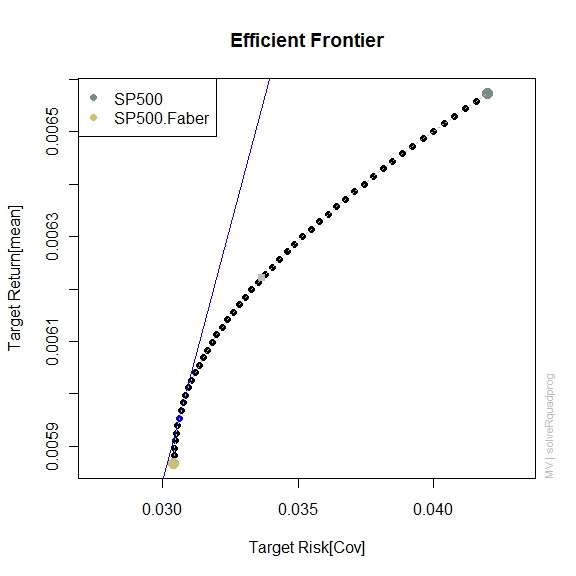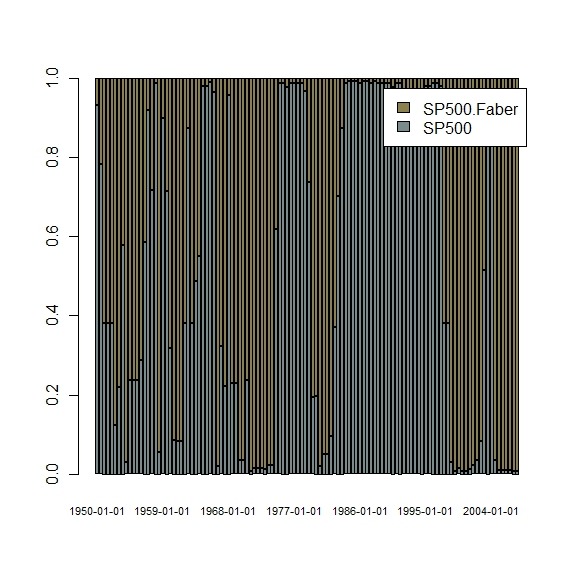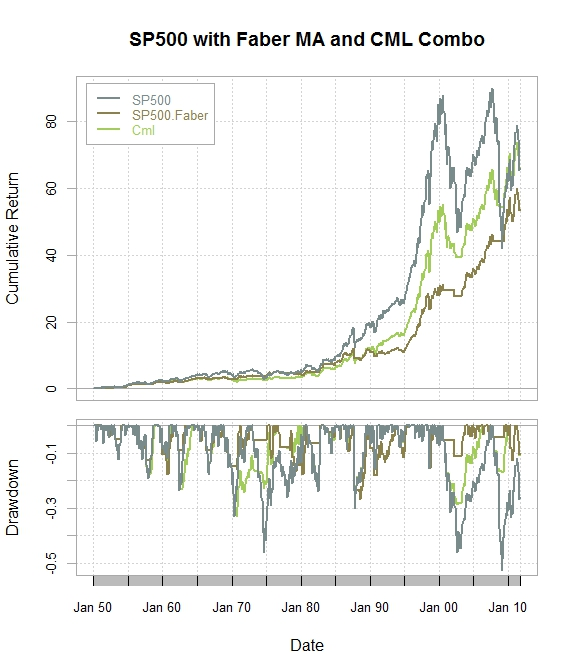Want to share your content on R-bloggers? click here if you have a blog, or here if you don't.

In my mind, there are two very disparate views in the money management space: Markowitz style diversification and Faber style tactical allocation.

I thought it would be fun to see what happens when we try to blend the two with an efficient frontier between two assets—”buy and hold” S&P 500 and a Faber 10-month tactical S&P 500 strategy.  In the process I will also introduce the fPortfolio package.

If we take the 10 month moving average S&P 500 systemFrom TimelyPortfolio

and treat it as a separate asset class, the efficient frontier since 1950 would look like this.From TimelyPortfolio

I think this is an interesting and unique way to look at it.  If we then take the rolling tangency portfolio the allocation would fluctuate and fairly closely mark bear and bull equity markets.From TimelyPortfolio

AS ALWAYS THIS IS NOT INVESTMENT ADVICE, JUST A FUN EXPERIMENT. SIGNIFICANT LOSSES ARE HIGHLY LIKELY IF YOU PURSUE THIS APPROACH.

The blended allocation looks fairly good.  There was no optimization or backtesting.  Please let me know if you discover improvements.From TimelyPortfolioFrom TimelyPortfolio

`require(quantmod)require(PerformanceAnalytics)   #get GSPC or S&P 500#feel free to change to whatever you would like#for non index do not include ^getSymbols("^GSPC",from="1896-01-01",to=Sys.Date())   #get monthly closesp500 <- to.monthly(GSPC)[,4]#do this to get from mmm yyyy to yyyy-mm-ddindex(sp500) <- as.Date(index(sp500))#get monthly returns from the monthly closes#multiple ways of doing thissp500.ret <- monthlyReturn(sp500)   #get 10 month Mebane Faber moving averagema <- runMean(sp500,n=10)#if close > 10 month moving average then 1 and 0 if  ma,1,0)   #merge originial return data with this new data#multiply the 1-month lagged signal by return#if signal is 0 then return is 0 indicating outreturnComp <- merge(sp500.ret,lag(signal,k=1)*sp500.ret)returnComp[is.na(returnComp[,2]),2] <- 0colnames(returnComp) <- c("SP500","SP500.Faber")   #jpeg(filename="performance summary.jpg",	quality=100,width=6, height = 7.5,  units="in",res=96)charts.PerformanceSummary(returnComp, ylog=TRUE,	colorset=c("lightcyan4","lightgoldenrod3"),	main="S&P 500 and Mebane Faber Moving Average System	Monthly Performance Since 1950")#dev.off()   #saved this require for later#since fPortfolio and f anything does not play well#with PerformanceAnalyticsrequire(fPortfolio)   #get frontier for the combination#of the original price and the Faber mov avg systemfrontier <- portfolioFrontier(as.timeSeries(returnComp))   #most of this comes directly from the fPortfolio demo#very slight changes have been made#we will run for the entire period#jpeg(filename="frontier plot.jpg",#	quality=100,width=6, height = 6,  units="in",res=96)#unfortunately title cannot be changed easilyfrontierPlot(frontier, pch=19, risk = "CVaR")minvariancePoints(frontier,pch=19,col="red")tangencyPoints(frontier,pch=19,col="blue")tangencyLines(frontier,pch=19,col="blue")equalWeightsPoints(frontier,pch=15,col="grey")singleAssetPoints(frontier,pch=19,cex=1.5,col=c("lightcyan4","lightgoldenrod3"))twoAssetsLines(frontier,lty=3,col="grey")legend("topleft",legend=colnames(returnComp),pch=19,col=c("lightcyan4","lightgoldenrod3"))#sharpeRatioLines(frontier,col="orange",lwd=2)#dev.off()   #now let's see what this looks like on a rolling basis#this from and to is not well documented in the fPortfolio#documentation so I hope it helps some people#will use 48 month rolling window and redo every 6 months#window needs to be large since Faber system will have lots of#0 returns, which can be handled by adding t-bill returns while outfrom <- rollingWindows(as.timeSeries(returnComp),period="48m",by="6m")\$fromto <- rollingWindows(as.timeSeries(returnComp),period="48m",by="6m")\$to   Spec = portfolioSpec()setTargetReturn(Spec) = mean(colMeans(as.timeSeries(returnComp)))Constraints = "LongOnly"#using Tangency but can also do Cml with rollingCmlPortfolio#or rollingMinvariancePortfoliorollTan <- rollingTangencyPortfolio(as.timeSeries(returnComp),Spec,Constraints,	from=from,to=to)#sapply works very nicely with the lists used in fPortfolio#get weights from each of the rolling periodstanweights <- sapply(rollTan,getWeights)rownames(tanweights) <- colnames(returnComp)   #jpeg(filename="rollling weight plot.jpg",	quality=100,width=6, height = 6,  units="in",res=96)barplot(tanweights,col=c("lightcyan4","lightgoldenrod4"),	legend.text=TRUE,names.arg=format(from,"%b %y"),	cex.names=0.7)#dev.off()   #do not know a slick way to do this#repeat the weights 6 times for the 6 month byfor (i in 1:NROW(t(tanweights))) {	for (j in 1:6) {		if (i==1 & j==1) {			tanweights.xts <- data.frame(t(tanweights[,i]))		} else {			#check to make sure we do not exceed number			#of rows in original return series - 48			#for the initialization			if (NROW(tanweights.xts) <= NROW(returnComp)-48)			tanweights.xts <- rbind(tanweights.xts,				data.frame(t(tanweights[,i])))		}	}}tanweights.xts <- xts(tanweights.xts,	order.by=index(returnComp)[48:NROW(returnComp)])   tanreturns <- lag(tanweights.xts,k=1)*returnComp[49:NROW(returnComp),]returnComp2 <- merge(returnComp,tanreturns[,1]+tanreturns[,2])colnames(returnComp2) <- c(colnames(returnComp)[1:2],"Cml")#jpeg(filename="risk return.jpg",	quality=100,width=6, height = 6,  units="in",res=96)chart.RiskReturnScatter(returnComp2)#dev.off()#since fPortfolio gives error on charts.PerformanceSummary#assemble quick one-pager#jpeg(filename="perf all.jpg",#	quality=100,width=6, height = 7,  units="in",res=96)layout(matrix(c(1, 2)), height = c(2.5,1.5), width = 1)par(mar = c(1, 4, 4, 2))chart.CumReturns(returnComp2,xaxis=FALSE,ylab="Cumulative Return",	colorset = c("lightcyan4","lightgoldenrod4","darkolivegreen3"),	main="SP500 with Faber MA and CML Combo",legend.loc="topleft")par(mar = c(5, 4, 0, 2))chart.Drawdown(returnComp2,main="",ylab="Drawdown",	colorset=c("lightcyan4","lightgoldenrod4","darkolivegreen3"))#dev.off()`